Courses

# Test: Casting Level - 2

## 20 Questions MCQ Test Manufacturing and Industrial Engineering | Test: Casting Level - 2

Description
This mock test of Test: Casting Level - 2 for Mechanical Engineering helps you for every Mechanical Engineering entrance exam. This contains 20 Multiple Choice Questions for Mechanical Engineering Test: Casting Level - 2 (mcq) to study with solutions a complete question bank. The solved questions answers in this Test: Casting Level - 2 quiz give you a good mix of easy questions and tough questions. Mechanical Engineering students definitely take this Test: Casting Level - 2 exercise for a better result in the exam. You can find other Test: Casting Level - 2 extra questions, long questions & short questions for Mechanical Engineering on EduRev as well by searching above.
QUESTION: 1

### Wood flour is added to core sand to improve:

Solution: Wood flour is added to improve collapsibility of core.

QUESTION: 2

### Which of the following sand ramming methods result into hardest layer at parting plane and around the pattern and less dense in top layers?

Solution: Jolting results in hardest layer at parting plane and around the pattern and less dense in top layer.

QUESTION: 3

### Recess provided in the material for locating and positioning of cores is called as:

Solution: Recess is provided in the material for locating and positioning of core is called Core print.

QUESTION: 4

Match the following according to the properties of moulding sand

List-I

a. Cohesiveness

c. Refractoriness

d. Collapsibility

List-II

i. Ability of breaking the mould with little force

ii. Ability to withstand higher temperature without losing strength

iii. Ability of bond formation of sand particles with other materials

iv. Ability to form bond between sand particles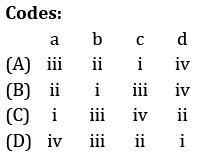Solution:
QUESTION: 5

Match the four basic elements of moulding sand with its percentage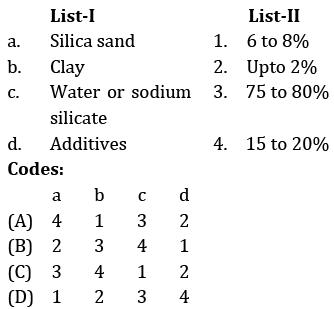Solution:
QUESTION: 6

Which one is true for strainer:

Solution: Strainer acts as a filter for separating the impurities present in molten metal.

QUESTION: 7

The shape factor for a casting in the form of an annular cylinder of outside diameter 30 cm, inside diameter 20 cm and height 30 cm (correction factor, k = 1 ) will be:

Solution: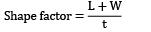L = height = 30cm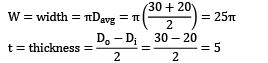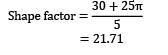QUESTION: 8

Match List-I (Type of casting) with List-II (Working principles) and select the correct answer using the codes given below the lists:

List-I

a. Die casting

b. Centrifugal casting

c. Centrifuging

d. Continuous casting

List-II

1. Molten metal is forced into the die under pressure

2. Axis of rotation does not coincide with axis of mould

3. Metal solidifies when mould is rotating

4. Continuously pouring molten metal into mould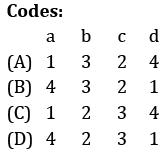Solution:

In die casting molten metal is forced in to die made up of metal.

In centrifugal casting axis of rotation coincide with axis of mould.

In centrifuging, axis of rotation does not coincide with axis of mould.

QUESTION: 9

The correct reasons for the occurrence of hot tear in casting among the following reasons will be:

i. Hindered contraction occurring immediately after metal has solidified

ii. Poor collapsibility of mould and core

iii. Too high pouring temperature

Solution:
QUESTION: 10

Directional solidification means:

Solution:

Directional solidification means solidification near riser will be at end.

Progressive solidification means the solidification is starting from wall of casting and proceeding towards the center of casting.

*Answer can only contain numeric values
QUESTION: 11

A steel slab casting of dimensions 30 cm x 30 cm x 6 cm with a side riser is to be casted horizontally into the mould. Using modulus method, the volume of the cylindrical riser is __________cm3

Solution:

The dimensions of the slab = 30 cm x 30 cm x 6 cm

This can be considered as a long bar with a cross section of (30 x 6)cm2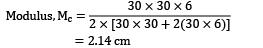MR = 1.2 Mc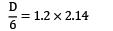D = 15.4 cm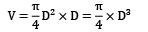= 2872.95 cm3

QUESTION: 12

The rate of formation of thickness (in mm) of the metal skin frozen during solidification of pure zinc casting is found to be 2 times of square root of solidification time (in s). What will be the solidification time if thickness of the solid zinc casting is 16 mm?

Solution: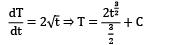Where T is thickness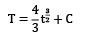At t = 0, T = 0 ⇒ C = 0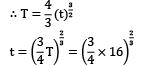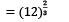= 5.24 sec

QUESTION: 13

In a gating design, sprue has an area 5.4cm2 , where the pouring basin meets the sprue, sprue length is 30 cm and desired molten metal flow rate is 900 cm3 /s. The area of the sprue at the bottom to avoid air aspiration effect is:

Solution: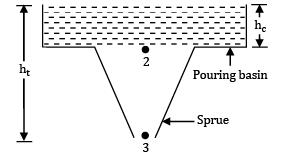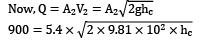hc = 14.16 cm

From continuity equation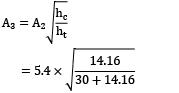= 3.0578 cm2

*Answer can only contain numeric values
QUESTION: 14

A cylindrical side riser is used for a sand casting mold. The casting itself is a rectangular plate with dimensions 7.5 x 12.5 x 2 cm3 . The solidification time for this casting is 1.6 min. The cylinder for the riser has a diameter to height ratio equal to 1. If the riser and casting are in same mold, then for a riser solidification time of 2 min, its dimension is __________ cm

Solution:

Volume of plate = 7.5 x 12.5 x 2 = 187.5 cm3

Surface area of plate

= 2 x (7.5 x 12.5 + 7.5 x 2 + 12.5 x 2)

=267.5 cm2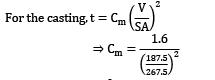Cm = 3.26 min/cm2

Now, we design the riser so that its solidification time is 2 min, using the same value of mold constant as they both are in the same mold.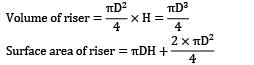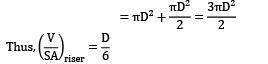Now, triser = 2 min (Given)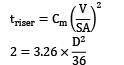D = 4.699 cm

*Answer can only contain numeric values
QUESTION: 15

The following data is observed for a casting process:

Mass of casting = 5000 kg

Density of metal = 8000 kg/m3

Pouring time = 10 sec

Efficiency factor = 0.9

The chock area of the casting is ___________mm2

Solution: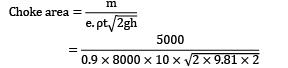= 0.01108595 m2

= 11085.95 mm2

QUESTION: 16

A cast steel slab of dimension 30 x 20 x 5 cm3 is poured horizontally using a side riser. The riser is cylindrical in shape with diameter and height both equal to 12 cm. The freezing ratio of mould for Caine’s empirical equation is:

Solution: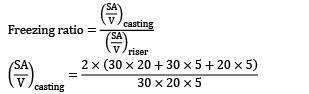= 0.567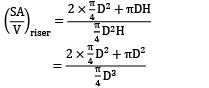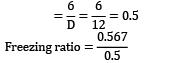= 1.134

QUESTION: 17

What is the modulus of a optimum side riser in the shape of square parallelopiped with side a:

Solution:

Optimum riser ⇒ heat transfer is minimum ⇒

S.A is minimum

Surface area = 2a2 + 4ah = S.A

V = a2 x h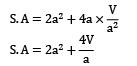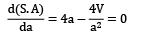⇒ 4V = 4a3

⇒ V = a3

⇒ a2h =a3 ⇒ a = h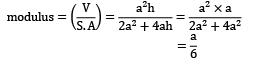QUESTION: 18

Following are the steps followed in a typical casting process:

i. Moulding

ii. Pattern making

iii. Melting and pouring

iv. Core making

v. Testing and inspection

vi. Fettling

The correct sequence of steps will:

Solution:

Correct sequence of steps in casting is Pattern making ➝ core making ➝ mould making ➝ melting and pouring ➝ fettling ➝ testing and inspection.

QUESTION: 19

In risering analysis, for finding the riser contribution, chills are used to:

Solution: In risering analysis, towards finding the riser contribution chills are used to increase riser contribution and increase edge distribution.

QUESTION: 20

Which of the following statements are true with regard to the hot chamber and cold chamber die cast machine?

i. Hot chamber die casting machine has a furnace for melting and holding the metal

ii. High melting temperature alloys of nonferrous type are best to die cast in hot chamber machines

iii. In cold chamber machine, metal is ladled by hand from a nearly holding furnace

Solution: